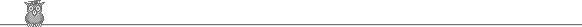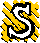### 42nd Putnam 1981A1.  Let the largest power of 5 dividing 112233 ... nn be 5f(n). What is limn→∞ f(n)/n2 ?A2.  We can label the squares of an 8 x 8 chess board from from 1 to 64 in 64! different ways. For each way we find D, the largest difference between the labels of two squares which are adjacent (orthogonally or diagonally). What is the smallest possible D?A3.  Evaluate: limk→∞ e-k ∫R (ex - ey) / (x - y) dx dy, where R is the rectangle 0 ≤ x, y, ≤ k.A4.  A particle moves in a straight line inside a square side 1. It is reflected from the sides, but absorbed by the four corners. It starts from an arbitrary point P inside the square. Let c(k) be the number of possible starting directions from which it reaches a corner after traveling a distance k or less. Find the smallest constant a2, such that from some constants a1 and a0, c(k) ≤ a2k2 + a1k + a0 for all P and all k.A5.  p(x) is a real polynomial with at least n distinct real roots greater than 1. [To be precise we can find at least n distinct values ai > 1 such that p(ai) = 0. It is possible that one or more of the ai is a multiple root, and it is possible that there are other roots.] Put q(x) = (x2 + 1) p(x) p'(x) + x p(x)2 + x p'(x)2. Must q(x) have at least 2n - 1 distinct real roots?A6.  A, B, C are lattice points in the plane. The triangle ABC contains exactly one lattice point, X, in its interior. The line AX meets BC at E. What is the largest possible value of AX/XE?B1.  Evaluate limn→∞ 1/n5 ∑ (5 r4 - 18 r2 s2 + 5 s4), where the sum is over all r, s satisfying 0 < r, s ≤ n.B2.  What is the minimum value of (a - 1)2 + (b/a - 1)2 + (c/b - 1)2 + (4/c - 1)2, over all real numbers a, b, c satisfying 1 ≤ a ≤ b ≤ c ≤ 4.B3.  Prove that infinitely many positive integers n have the property that for any prime p dividing n2 + 3, we can find an integer m such that (1) p divides m2 + 3, and (2) m2 < n.B4.  A is a set of 5 x 7 real matrices closed under scalar multiplication and addition. It contains matrices of ranks 0, 1, 2, 4 and 5. Does it necessarily contain a matrix of rank 3?B5.  f(n) is the number of 1s in the base 2 representation of n. Let k = ∑ f(n) / (n + n2), where the sum is taken over all positive integers. Is ek rational?B6.  Let P be a convex polygon each of whose sides touches a circle C of radius 1. Let A be the set of points which are a distance 1 or less from P. If (x, y) is a point of A, let f(x, y) be the number of points in which a unit circle center (x, y) intersects P (so certainly f(x, y) ≥ 1). What is sup 1/|A| ∫A f(x, y) dx dy, where the sup is taken over all possible polygons P?To avoid possible copyright problems, I have changed the wording, but not the substance, of all the problems. The original text and official solutions were published in American Mathematical Monthly 89 (1982) 681. They are also available (with the solutions expanded) in: Gerald L Alexanderson et al, The William Lowell Putnam Mathematical Competition, 1965-1984. Out of print, but available in some university libraries.

Putnam home

© John Scholes
jscholes@kalva.demon.co.uk
4 Sep 1999